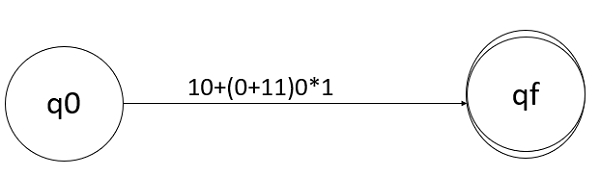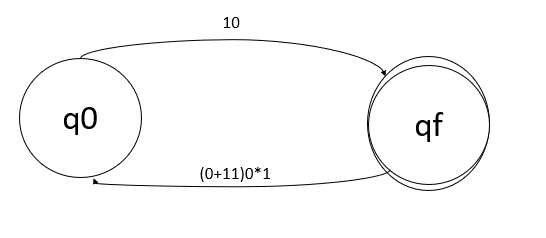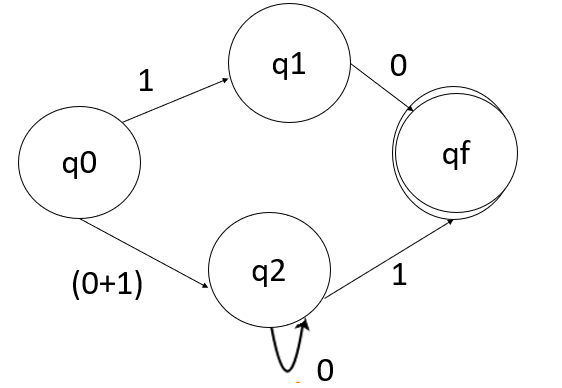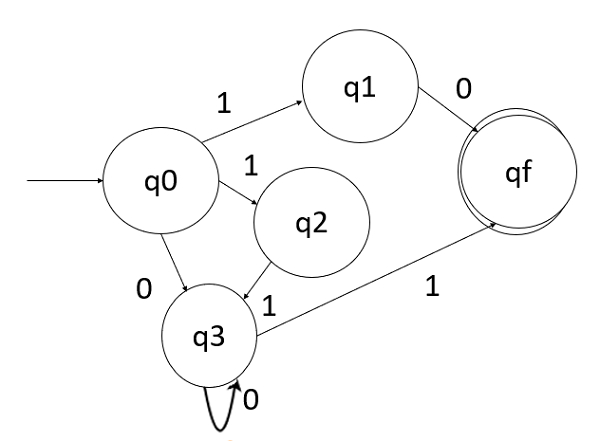# Design finite automata from a given regular expression.

Finite automata (FA) is an abstract computing device. It can be represented in the following −

• Graphical (Transition diagrams)
• Tabular (Transition table)
• Mathematical (Transition function)

The formal definition of FA is that it is a five tuples.

M=(Q, Σ, δ, q0, F)

Where,

• Q: Finite set called states
• Σ: Finite set called alphabets
• δ: Q × Σ → Q is the transition function
• q0 ∈ Q is the start or initial state
• F: Final or accept state

## Regular expression

The regular expression can be used to describe a sequence of patterns that defines a string. It is used to match character combinations in strings.

The language accepted by some regular expression are referred to as regular language

Now let’s consider RE 10+(0+11)0*,

Construct finite automata for this regular expression −

### Step 1Initially consider two states q0 and qf. Also, consider the initial and final states, q0 to qf and the given regular expression 10+(0+11)0*1

### Step 2Now applying the union operation, q0 on 10 goes to qf and q0 on (0+11)0*1 goes to qf.

### Step 3Now, divide the expression by applying concatenation i.e. let L1=0, L2=1. Concatenate both and we get L= L1.L2.

Applying this formula on regular expression, so q0 on 1 goes to q1 and q1 on 0 goes to qf. Similarly, q0 on (0+1) goes to q2 and q2 on 0*1 goes to qf.

### Step 4q0 on 1 goe to q1 and q1 on 0 goes to qf, q0 on (0+1) goes to q2, q2 on 0 goes to q2 itself and on 1 goes to qf.

### Step 5

The final finite automata for the given regular expression is as follows −